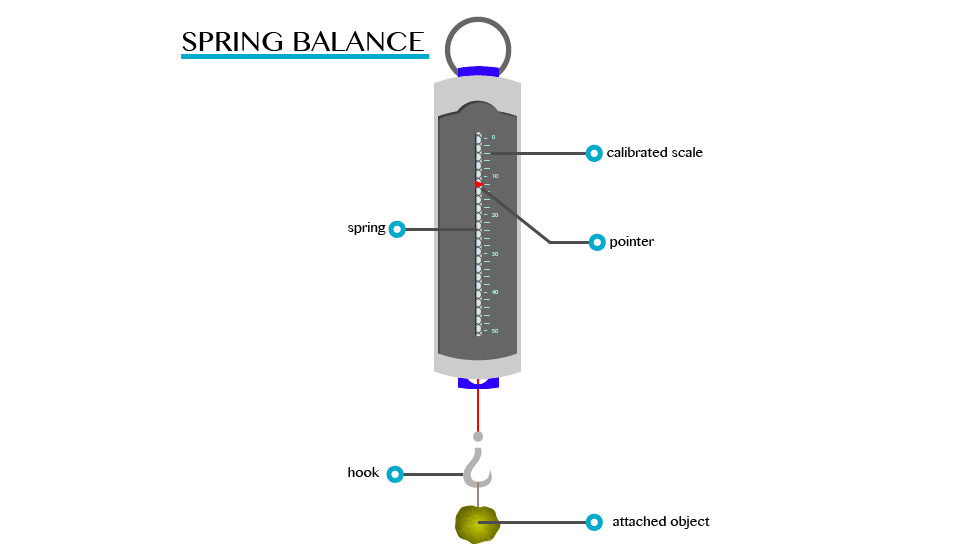Home PHYSICS TOPIC 4: FORCE | PHYSICS FORM 1

TOPIC 4: FORCE | PHYSICS FORM 1

66
0
SHAREForce

A force is an interaction that causes a change. In mechanics, a force is an interaction that causes a change in velocity (an interaction that causes acceleration).
The Concept of Force
Explain the concept of force
For better understanding of this concept, let’s ask ourselves the following question:
1. What causes/makes a body at rest to move?
2. What causes the same body in motion to stop?
The
answers to these questions, is of course that a force is required to do
so. Here, an applied force to a body can cause the body at rest to move
or if already moving a body application of force can do the following
when applied to a body.
1. Force can cause a change in the way the object moves
2. Change its size or shape
3. Change the direction in which an object is moving
A
force is defined as a push or pulls experienced by an object. The force
is usually described in terms of its size (magnitude) and direction.
The S.I Unit of Force
State the S.I unit of force
The SI unit of force is a Newton (N).
A Newton (1N) can be defined as the amount of force required to give a mass of one kilogram (1kg) an acceleration of 1m/s2
Measuring Instrument (Device)
The instrument that is used to measure the amount of force that is exerted on an object is called spring balance.
A
spring balance can be used to measure small forces. It consists of a
coiled spring fixed to the other end with a hook at the other end. The
body upon which the force acts is attached to the hook. The distance
through which the spring is stretched is directly proportional to the
force applied by the balance.
A pointer is attached to the spring and the force is indicated on a calibrated scale.SHARE
Previous articleTOPIC 3: MEASUREMENT | PHYSICS FORM 1